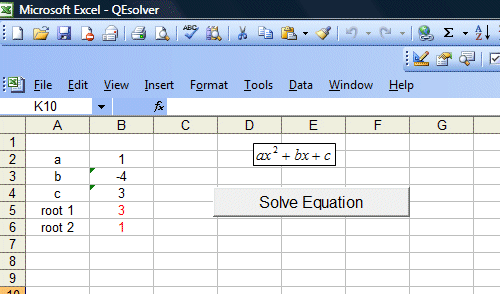[Back to Excel VBA Sample Code]

We have presented to you a quadratic equation solver in the Visual Basic 6 Tutorial. You can also create a similar program using MS Excel Editor. In fact, it is easier to do it in MS Excel as you just enter the values into the cells rather than having to create the text boxes. So for those of you who are without a copy of MS Visual Basic compiler, but you have MS Office, you can copy the code and try this program out in your MS Excel

 The Code: Private Sub CommandButton1_Click() Dim a, b, c, det, root1, root2 As Single a = Cells(2, 2) b = Cells(3, 2) c = Cells(4, 2) det = (b ^ 2) - (4 * a * c) If det > 0 Then root1 = (-b + Sqr(det)) / (2 * a) root2 = (-b - Sqr(det)) / (2 * a) Cells(5, 2) = Round(root1, 2) Cells(6, 2) = Round(root2, 2) ElseIf det = 0 Then root1 = (-b) / 2 * a Cells(5, 2) = root1 Cells(6, 2) = root1 Else Cells(5, 2) = "No root" End If End Sub

Explanation:

The format of the quadratic equation  is as below:

ax2+bx+c  , where a,b,c are constants.

The number of roots depends on the determinant of b2-4ac

If b2-4ac>0  then there are two roots

If b2-4ac=0  then there is only one root

If b2-4ac<0  then there is no root.

By making use the above conditions and employ the use of If....Then...Else statements, the program is able to solve the quadratic equation. The output is as shown in Figure 12.1Figure 12.1